Fracture simulations and applications

How to use RGeostats package step-by-step.

Fracture simulations and applications

Simulation domain and fracture parameters

The first task is to create the new element modF belonging to the fracture class which contains the definition of the fractures.
It is constituted of two families of fractures (the description of their spatial characteristics can be obtained by checking the on-lline help of fracture.create function)
Case without main fault
Code: Select all
`modF <- fracture.create(xmax=80,ymax=10,deltax=10,deltay=100,mean=2,stdev=0.6)modF <- fracture.create(fracture=modF,flag.family=T,orient=10,dorient=20,                        theta0=0.6,alpha=0.5,ratcst=0.2,prop1=0.5,prop2=0.5,                        aterm=10,bterm=10,range=0.5,flag.fault=F)modF <- fracture.create(fracture=modF,flag.family=T,orient=-20,dorient=5,                        theta0=0.6,alpha=0.5,ratcst=0.2,prop1=0.5,prop2=0.5,                        aterm=100,bterm=100,range=0,flag.fault=F)`

Now that the fracture definition is available, we can run the first simulation to generate a stochastic outcome of the fractures.
It is simply dependent on the seed which serves for the generation of the set of random numbers. The resulting object simF belongs to the fracsim class.
Code: Select all
`seed = 918273                                     simF <-fracture.simulate(modF,save=T,draw=T,seed=seed,asp=1,                         title=paste0("Simulation; seed=",seed,sep=""))`

The next figure illustrates the simulation of the set of fractures.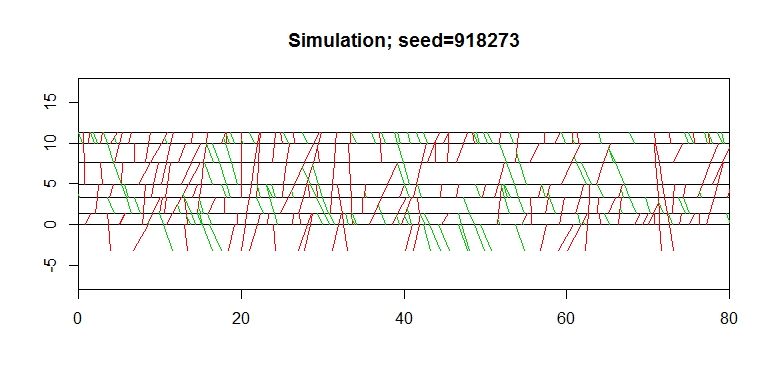With main fault

In this section, we add the presence of a main deterministic fault. This fault serves as an attraction to the fractures of the different families.
Code: Select all
`  modF <- fracture.create(fracture=modF,flag.fault=T,                        coord=40,orientf=0,thetal=5,rangel=5,thetar=5,ranger=10)`

We are now ready to run another simulation of the fractures in presence of the main fault this time.
Code: Select all
`seed = 654321simF <- fracture.simulate(modF,save=T,draw=T,seed=654357,asp=1,                          title=paste0("Simulation; seed=",seed,sep=""))`

The next figure represents the simulation in presence of the main Fault.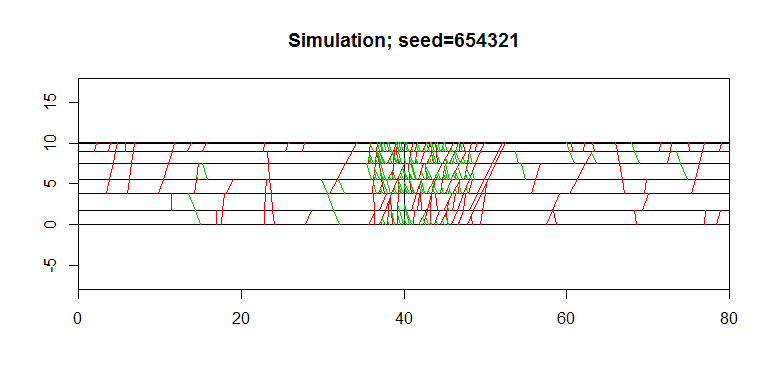Another simulation with same layers
The feature demonstrated here consists in retrieving the set of layers generated previously and in simulating another set of fractures.
Code: Select all
`seed = 981255banc = simF\$elevationssimF <- fracture.simulate(modF,save=T,draw=T,seed=seed,asp=1,elevations=banc,                          title=paste0("Simulation; seed=",seed,sep=""))`

Here is the second set of fractures simulated using the same set of layers as before.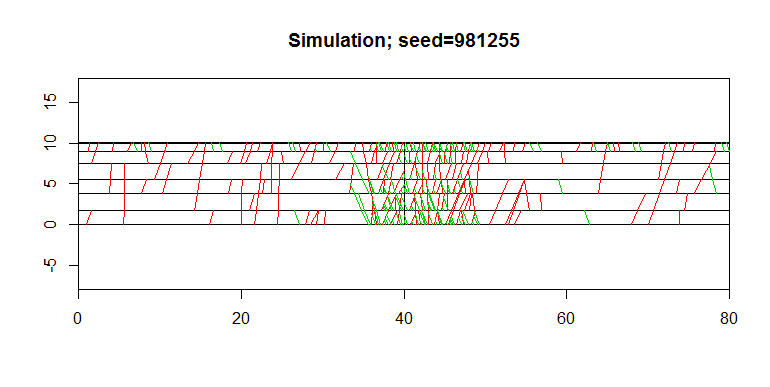Fracture lengthes and spacing
The feature exploited here allows extracting various characteristics from the object simF where the simulated fractures have been stored. It allows in particular to establish the histogram of fracture lengths or of the spacing between consecutive fractures at the first layer rank, per family.
Code: Select all
`numbanc=length(banc)/2for (ifam in 1:simF\$nfamilies) {  long = fracsimu.extract(simF,mode="length", family=ifam,layer.rank=numbanc)  hist(long,nclass=100,freq=F, main=paste("Length Family F",ifam,sep=""))  interd = fracsimu.extract(simF, mode="dist", family=ifam, layer.rank=1)  xlab=""  xlab=paste0("F ",ifam," Nb=",              length(interd)," Min=",round(min(interd),3)," Max=",              round(max(interd),3)," Mean=",round(mean(interd),3))  hist(interd,nclass=10,freq=F, main=paste("Spacing Family F",ifam,sep=""),xlab=xlab)}`

The following figures represent the histograms of the fracture lengths and the fracture spacing for the first two families.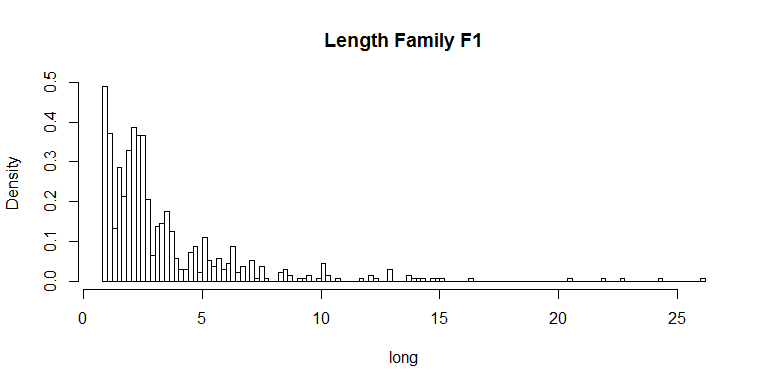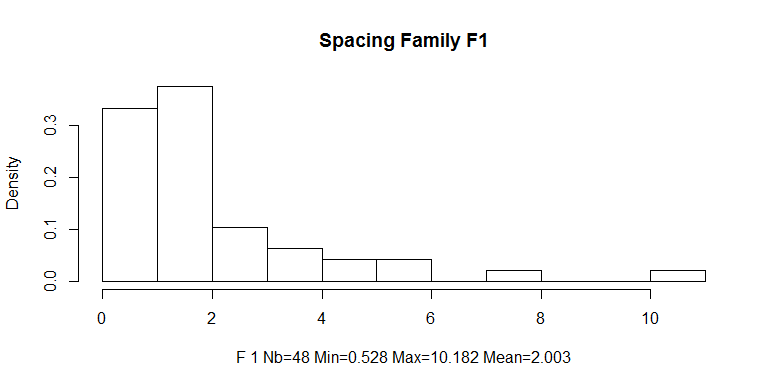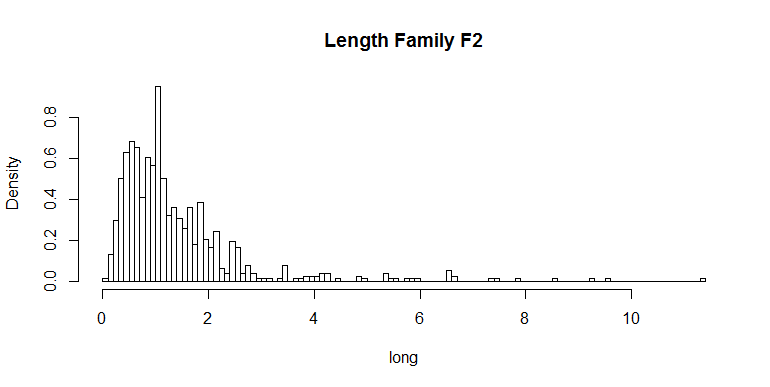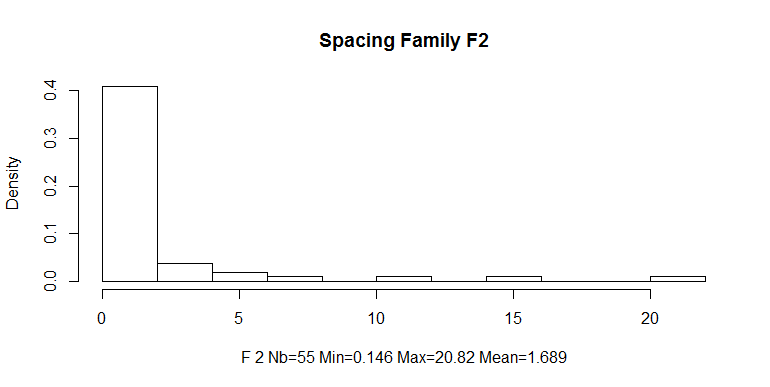Permeabilities bloc at 25cm meshes
We now plunge the set of simulated fractures in a set of fine block cells. First we define this set of cells.
Code: Select all
`              mesh    = 0.25nvert   = simF\$ymax/meshnhor    = simF\$xmax/meshbloc0   = db.create(x0=c(0,0),nx=c(nhor,nvert),dx=c(mesh,mesh))bloc0   = db.add(bloc0,facies=1)`

Now we can launch the calculation of the permeabilities induced by the simulated fractures within the cells. The permeability results of the 3 types of elements: the permeability carried by the matrix (Kmat), the permeability assigned to the inter-layer surfaces (Kbench) and finally the permeability attached to the main Fault and the two fracture families.(tabperm).
Code: Select all
`Kmat    = 1Kbench  = 1tabperm = c(100,100,10)bloc0  <- fracsimu.to.block(simF,bloc0,                            permmat=Kmat,permbench=Kbench,permtab=tabperm)plot(bloc0,asp=1,col=terrain.colors(6),flag.log10=T     title="Permeabilities in Grid cells")`

The next figure shows the permeabilities attached to each cell of the block system. For better legibility, the color scale is log10-transformed.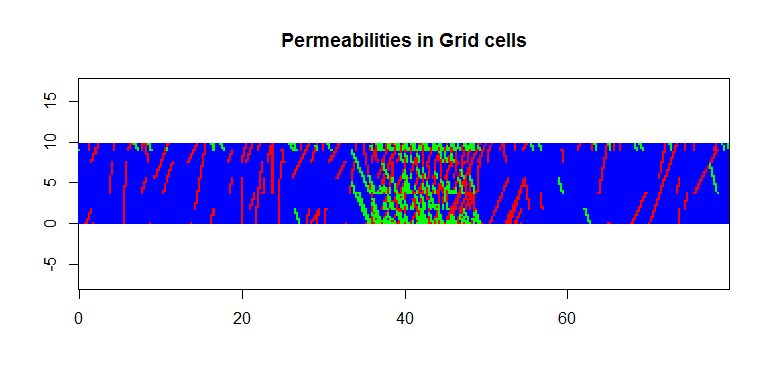Upscaling at 2m meshes
In this paragraph, we upscale the permeabilities from the fine cells (25cm edge) to a set of much larger cells (2m edge). The larger block system is created first.
Code: Select all
`mesh   = 2nvert  = simF\$ymax/meshnhor   = simF\$xmax/meshbloc1  = db.create(x0=c(0,0),nx=c(nhor,nvert),dx=c(mesh,mesh))`

We can now perform the upscaling procedure both along X and Y.
Code: Select all
`bloc1  = db.upscale(bloc0,bloc1,orient=1,"UpX")bloc1  = db.upscale(bloc0,bloc1,orient=2,"UpY")plot(bloc1,name="UpX.Frac2Block.Perm.mean",asp=1,     title = "Permeability Upscaled for X-Flow")plot(bloc1,name="UpY.Frac2Block.Perm.mean",asp=1,     title = "Permeability Upscaled for Y-Flow")`

The next two figures represent the mean upscaled permeabilities.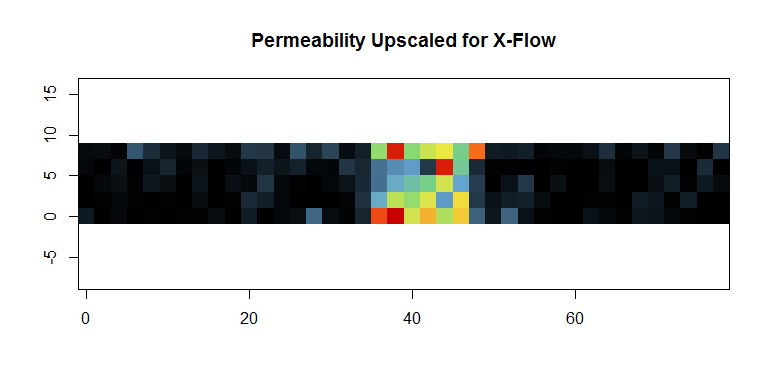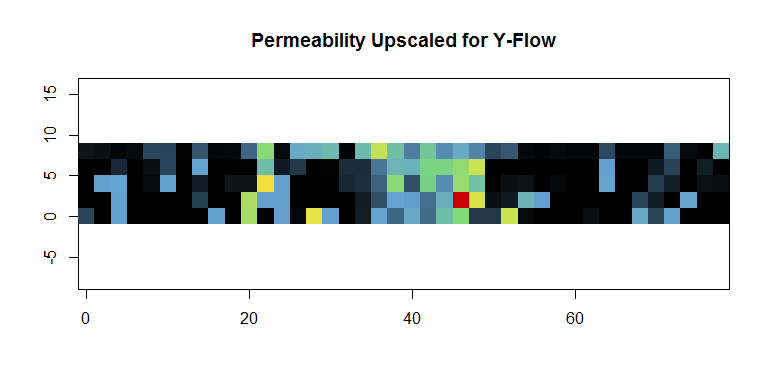Variogram of the upscaled permeabilities along X
In the current paragraph, we quantify the spatial characteristics of the permeability upscaled along the X axis. We calculate the experimental variogram and fit a model using the automatic procedure.
Code: Select all
`bloc1 = db.locerase(bloc1,"z")bloc1 = db.locate(bloc1,"UpX.Frac2Block.Perm.mean","z")varioupX = vario.grid(bloc1,dirincr=matrix(c(1,0),byrow=1),nlag=c(20))vmodel   = model.auto(varioupX,draw=F)xlab=""for (i in 1:vmodel\$ncova){  range = vmodel[i]\$range  vart  = vmodel\$basics[[i]]\$vartype  sill  = vmodel[i]\$sill  xlab  = paste0(xlab,vart,"S:",round(sill,3),"R:",round(range,0),sep=" ")}plot(varioupX,npairdw=1,inches=0.05,lty=2,lwd=2,     title = "Variogram along X for Permeability upscaled for X-Flow")model.plot(vmodel,vario=varioupX,add=T,sub=xlab,font.sub=1.5)`

The next figure represents the experimental variogram of the upscaled permeability together with the model fitted automatically.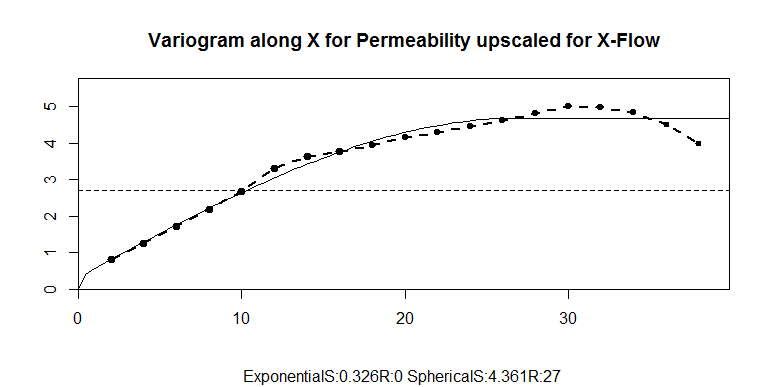Extract fault intersections along well
When providing a well trajectory (well.line) we can extract the set of fractures intercepted by this broken line. They are stored in the table well.found.
Code: Select all
`well.line  <- matrix(c(0,10,10,5,60,5,80,0),nrow=4,byrow=T)well.found <- fracsimu.to.well(simF,well.line,permtab=tabperm)plot(simF,asp=1, title="Fractures and Well")lines(well.found[,1:2],col="black",pch=4)points(well.found[,1:2],col="blue",pch=19)`

The next figure represents the set of simulated fractures and overlays the extracted set of fractures intercepted by the well trajectory.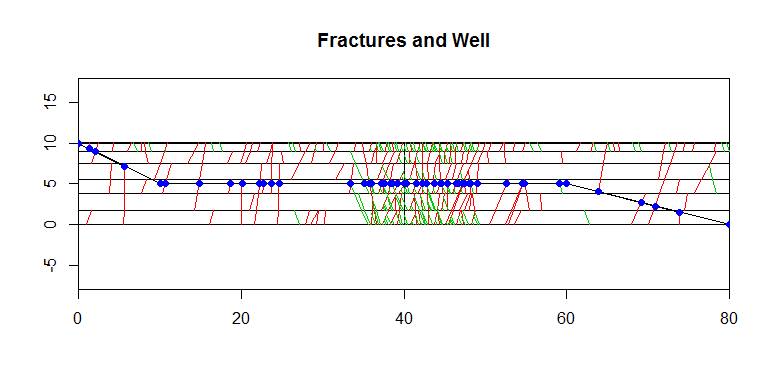Plunge the well into the bloc0 and fluid propagation
We now consider that the fluid is injected in the block via the well. The fluid then penetrates the block system with speeds which depend upon the permeability: the larger the permeability, the faster the penetration.
Code: Select all
` bloc0 <- fracsimu.well.to.block(bloc0,simF,well.found,                                name.perm="Frac2Block.Perm",radix="wini")bloc0 <- fluid.propagation(bloc0,facies="facies",fluid="wini.Fluid",                           perm="wini.Perm",nfacies=1,nfluids=1,                           seed=12345,radix="Ecoulini",verbose=F)zmax=max(bloc0[,9],na.rm=T)/4plot(bloc0,col=rainbow(20,start=0.2),name="Ecoulini.Fill.Date",asp=1,zlim=c(0,zmax),     title=paste("Propagation using initial permeabilities - iteration:",zmax) )`

In the next figure the fluid penetration has been interrupted (after a given time interval) and the result is represented.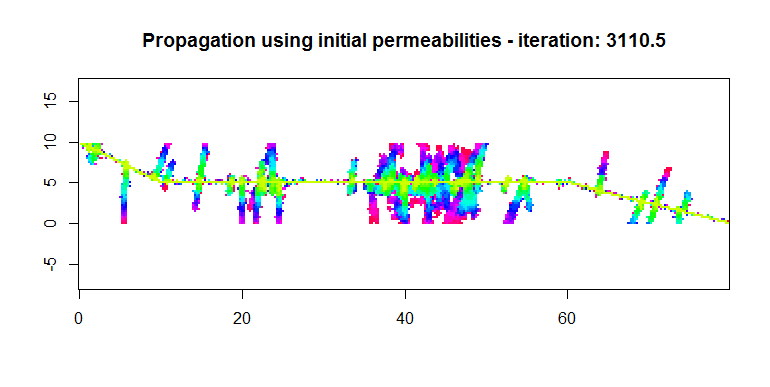Modified permeabilities at well and fluid propagation
We now imagine that the injection well provokes some perturbation to the permeabilities in the vicinity of the penetration. This perturbation is defined per family. It is characterized by the value at the well interception and the distance at which this perturbation vanishes (exponentially).
Code: Select all
`for(i in 1:length(well.found[,5])) {  if (well.found[i,5]==1) {well.found[i,6]=1000; well.found[i,8]=5}  if (well.found[i,5]==2) {well.found[i,6]=0;    well.found[i,8]=10}}`

The new injection well is plunged in the block system and the fluid propagation is performed again.
Code: Select all
`bloc0 <- fracsimu.well.to.block(bloc0,simF,well.found,                                name.perm="Frac2Block.Perm",radix="wmod")bloc0 <- fluid.propagation(bloc0,facies="facies",fluid="wmod.Fluid",                           perm="wmod.Perm",nfacies=1,nfluids=1,                           seed=12345,radix="Ecoulmod",verbose=F)`

Plunge the well into the bloc0 and fluid propagation
Code: Select all
`plot(bloc0,col=rainbow(20,start=0.2),name="Ecoulmod.Fill.Date",asp=1,zlim=c(0,zmax),     title=paste("Propagation using modified permeabilities - iteration:",zmax))`

By comparing this figure to the previous one, we can appreciate the impact of the perturbation in the fluid propagation.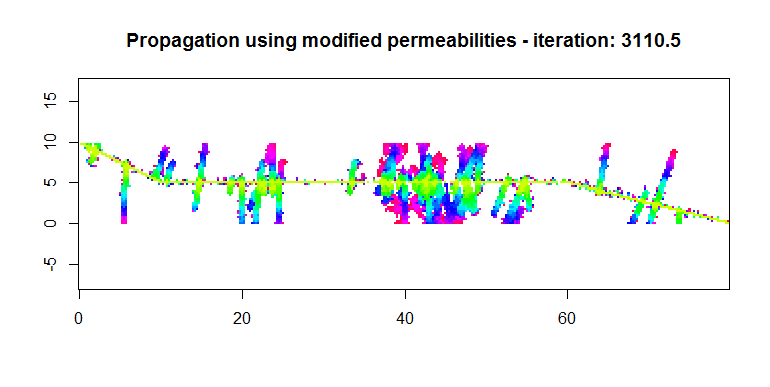Compute the size of the blocks of non damaged matrix
First draw the blocks of matrix , bench surfaces and fractures being the borders.
Code: Select all
`Kmat    =1Kbench  = 2tabperm = c(3,3,3)bloc0  <- fracsimu.to.block(simF,bloc0,                            permmat=Kmat,permbench=Kbench,permtab=tabperm,radix="matrix")plot(bloc0,asp=1,col=terrain.colors(3),     title="Block borders")`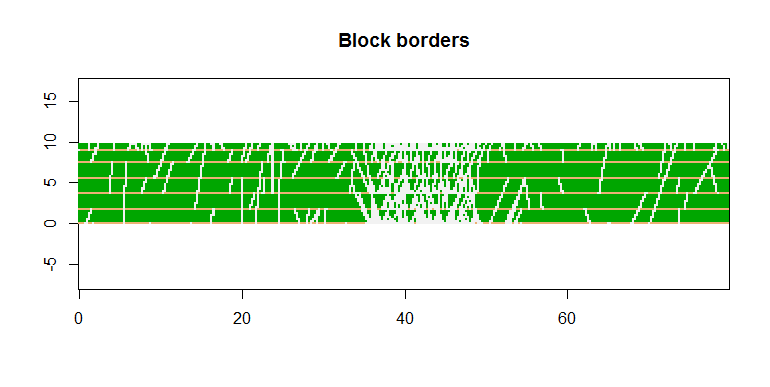Compute the connected components ("grains" correspond to the matrix valuated to 1)
Code: Select all
`compoc=morpho(bloc0,vmin=0.5,vmax=1.5,oper="cc")unique(compoc[,"cc.matrix.Perm"])range(compoc[,"cc.matrix.Perm"],na.rm=T)plot(compoc,zlim=c(1,max(compoc[,"cc.matrix.Perm"],na.rm=T)),col=rainbow(20,start=0.2),     pos.legend=4,name="cc.matrix.Perm",title="Connected components blocks")`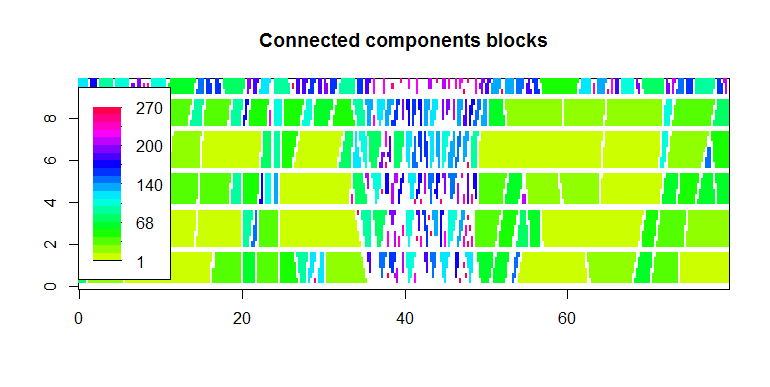Draw the histogram of the connected component sizes.
Code: Select all
`hist(compoc[,7],main="Connected components blocks")`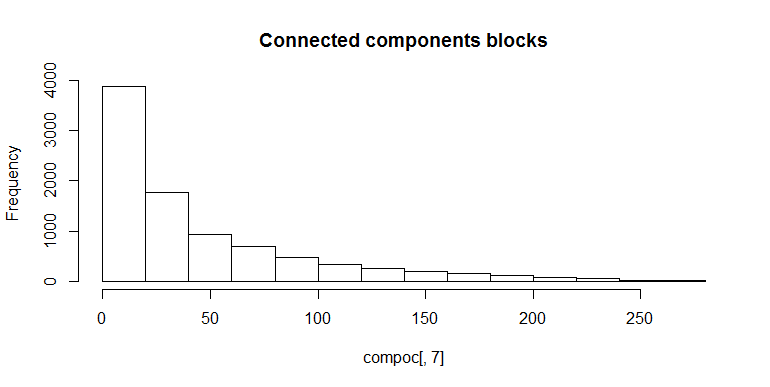helene beucher

Posts: 6
Joined: Fri Sep 21, 2012 3:50 pm